Next: Conic sections Up: Useful mathematics Previous: Precession

# Curvilinear coordinates

In the cylindrical coordinate system, the standard Cartesian coordinatesand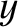are replaced by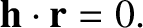and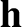. Here,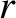is the perpendicular distance from the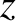-axis, and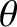the angle subtended between the perpendicular radius vector and the-axis. (See Figure A.2.) A general vector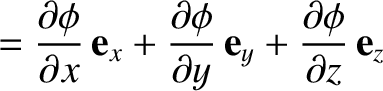is thus written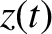(A.97)

where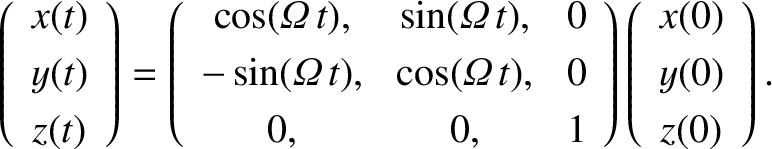and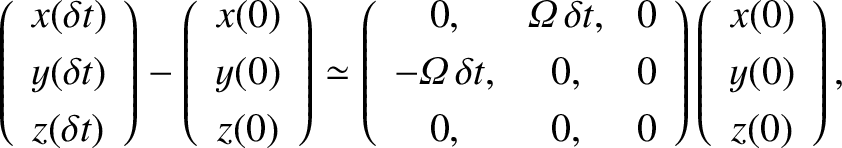. (See Figure A.2.) The unit vectors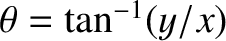,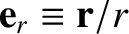, and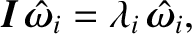are mutually orthogonal. Hence,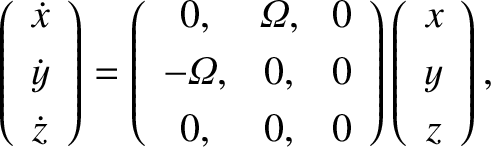, and so on. The volume element in this coordinate system is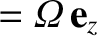. Moreover, the gradient of a general scalar field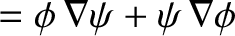takes the form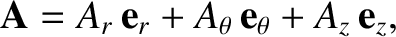(A.98)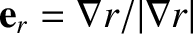In the spherical coordinate system, the Cartesian coordinates,, andare replaced by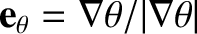,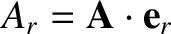, and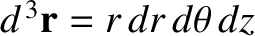. Here,is the radial distance from the origin,the angle subtended between the radius vector and the-axis, and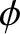the angle subtended between the projection of the radius vector onto the-plane and the-axis. (See Figure A.3.) Note thatandin the spherical system are not the same as their counterparts in the cylindrical system. A general vectoris written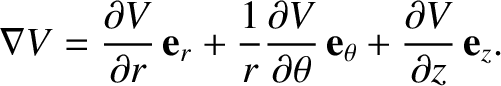(A.99)

where,, and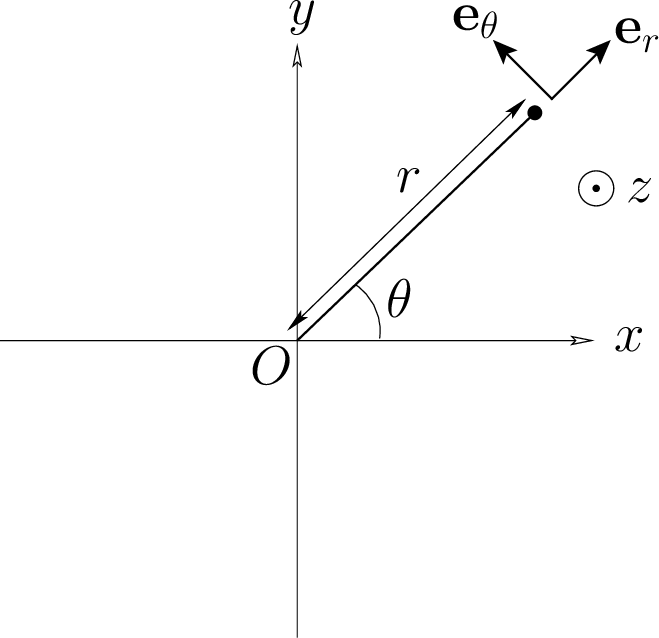. The unit vectors,, and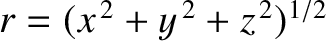are mutually orthogonal. Hence,, and so on. The volume element in this coordinate system is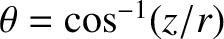. Moreover, the gradient of a general scalar fieldtakes the form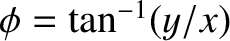(A.100)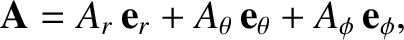Next: Conic sections Up: Useful mathematics Previous: Precession
Richard Fitzpatrick 2016-03-31